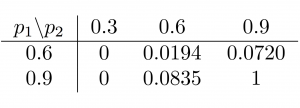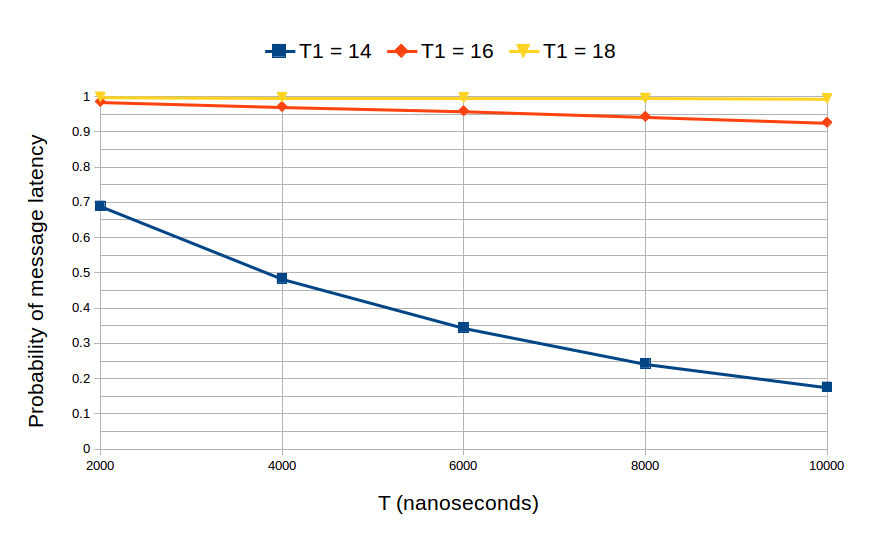# Producer and Consumer

1. Description

This example illustrates how designers can create hierarchical channels that encapsulate both design structure and communication protocols. In the design, once a nanosecond the producer will write one character to the FIFO with probability p1, while the consumer will read one character from the FIFO with probability p2. The FIFO which is derived from sc_channel encapsulates the communication protocol between the producer and the consumer.

2. Implementation

The FIFO channel is designed to ensure that all data is reliably delivered despite the varying rates of production and consumption. The channel uses an event notification hanshake protocol for both the input and output. It uses a circular buffer implemented within a static array to store and retrieve the items within the FIFO. We assume that the sizes of the messages and the FIFO buffer are fixed. Hence, it is obvious that the time required to transfer completely a message, or message latency, depends on the production and consumption rates, the FIFO buffer size, the message size, and the probabilities of successful writing and reading. The probabilities of writing and reading are implemented with the Bernoulli distributions with probabilities p1 and p2 respectively from GNU Scientific Library (GSL).

Source code and automated shell scripts – producer_consumer.tar.gz

3. Experimental results

The quantitative analysis under consideration is: “What is the probability that messages are transfered completely within T1 nanoseconds during T nanoseconds of operation?”. This kind of analysis can, thus, be conducted in the early design steps. To formulate the underlying property more precisely, we have to take into account the agreement protocol between the producer and consumer, i.e., the simple protocol can be every message has special starting delimiter with the character ‘&’ and ending delimiter with the character ‘@’. Thus, the property can be translated in BLTL as follows:

G <= T ((c_read =  ‘&’) => F <= T1 (c_read = ‘@’))

where c_read is the character read in the FIFO by the consumer.

We want to compute the probability that the following property satisfies every 1 nanosecond, with the absolute error 0.02 and the level of confidence 98%. In this verification, both the FIFO buffer size and message size are 10 characters including the starting and ending delimiters, and the production and consumption rates are 1 nanosecond. First, we check this property with the various values of p1 and p2. The results are given in the following table with T = 5000 and T1 = 25 nanoseconds. It is trivial that the probability that the message latency is smaller than T1 time increases when p1 and p2 increase. That means that, in general, the latency is shorter when the either the probability that the producer successfully writes to the FIFO increases, or the probability that the consumer successfully reads from the FIFO increases.Second, we compute the probability that a message is sent completely (or the message latency) from the producer to the consumer within T1 time over a period of T time of operation, in which the probabilities p1 and p2 are fixed at 0.9. The following figure shows this probability with different values of T1 over T = 10000 nanoseconds. It is observed that the message latency is almost smaller than 18 nanoseconds.